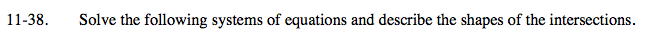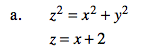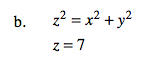### Home > A2C > Chapter 11 > Lesson 11.2.1 > Problem11-38

11-38.Substitute the second equation for z in the first, and simplify to find an equation of x and y.

What kind of graph is this?

$x =\frac{1}{4}y^2 -1$See part (a).